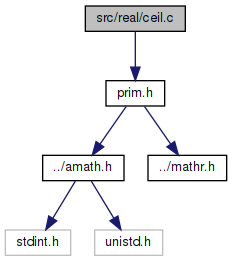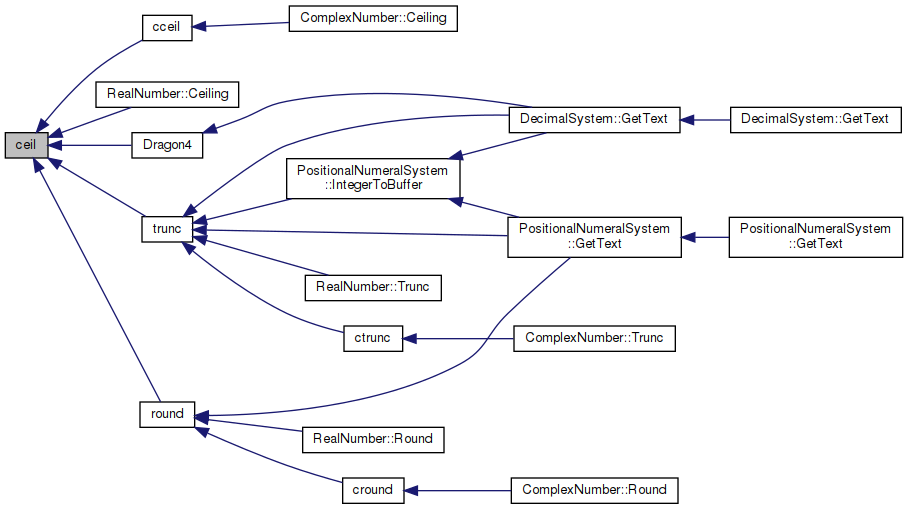amath  1.8.5 Simple command line calculator
ceil.c File Reference

Ceiling function. More...

`#include "prim.h"`
Include dependency graph for ceil.c:Go to the source code of this file.

## Functions

double ceil (double x)
Ceiling function. More...

## Variables

static const double huge = 1.0e300

## Detailed Description

Ceiling function.

Definition in file ceil.c.

## ◆ ceil()

 double ceil ( double x )

Ceiling function.

Parameters
 x
Returns
x rounded toward -inf to integral value
```Method
Bit twiddling```
```Exception
Inexact flag raised if x not equal to ceil(x).
```

Definition at line 63 of file ceil.c.

References huge.

Referenced by cceil(), RealNumber::Ceiling(), Dragon4(), round(), and trunc().

64 {
65  int32_t i0,i1,j0;
66  uint32_t i,j;
67  EXTRACT_WORDS(i0,i1,x);
68  j0 = ((i0>>20)&0x7ff)-0x3ff;
69  if(j0<20) {
70  if(j0<0) { /* raise inexact if x != 0 */
71  if(huge+x>0.0) { /* return 0*sign(x) if |x|<1 */
72  if(i0<0) {
73  i0=0x80000000;
74  i1=0;
75  }
76  else if((i0|i1)!=0) {
77  i0=0x3ff00000;
78  i1=0;
79  }
80  }
81  } else {
82  i = (0x000fffff)>>j0;
83  if(((i0&i)|i1)==0) return x; /* x is integral */
84  if(huge+x>0.0) { /* raise inexact flag */
85  if(i0>0) i0 += (0x00100000)>>j0;
86  i0 &= (~i);
87  i1=0;
88  }
89  }
90  } else if (j0>51) {
91  if(j0==0x400) return x+x; /* inf or NaN */
92  else return x; /* x is integral */
93  } else {
94  i = ((uint32_t)(0xffffffff))>>(j0-20);
95  if((i1&i)==0) return x; /* x is integral */
96  if(huge+x>0.0) { /* raise inexact flag */
97  if(i0>0) {
98  if(j0==20) i0+=1;
99  else {
100  j = i1 + (1<<(52-j0));
101  // NOTICE: Is this a correct cast?
102  if((int32_t)j<(int32_t)i1) i0+=1; /* got a carry */
103  i1 = j;
104  }
105  }
106  i1 &= (~i);
107  }
108  }
109  INSERT_WORDS(x,i0,i1);
110  return x;
111 }
#define INSERT_WORDS(d, ix0, ix1)
Set a double from two 32 bit ints.
Definition: prim.h:187
static const double huge
Definition: ceil.c:48
#define EXTRACT_WORDS(ix0, ix1, d)
Get two 32 bit ints from a double.
Definition: prim.h:156
Here is the caller graph for this function:## ◆ huge

 const double huge = 1.0e300
static

Definition at line 48 of file ceil.c.

Referenced by ceil().